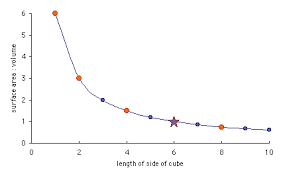## How to Calculate and Solve for Volume Ratio | Design of Gating SystemThe image above represents volume ratio.

To compute for volume ratio, two essential parameters are needed and these parameters are Mass of the Liquid Metal filling the Mould Cavity, Gates and Risers (G) and Casting Volume (V).

The formula for calculating volume ratio:

Kv = G / V

Where:

Kv = Volume Ratio
G = Mass of the Liquid Metal Filling the Mould Cavity,Gates and Risers
V = Casting Volume

Let’s solve an example;
Find the volume ratio when the mass of the liquid metal filling the mould cavity, gates and risers is 12 and the casting volume is 24.

This implies that;

G = Mass of the Liquid Metal Filling the Mould Cavity,Gates and Risers = 12
V = Casting Volume  = 24

Kv = G / V
Kv = 12 / 24
Kv = 0.5

Therefore, the volume ratio is 0.5.

Calculating the Mass of the Liquid Metal Filling the Mould Cavity, Gates and Risers when the Volume Ratio and the Casting Volume is Given.

G = Kv x V

Where;

G = Mass of the Liquid Metal Filling the Mould Cavity,Gates and Risers
Kv = Volume Ratio
V = Casting Volume

Let’s solve an example;
Find the mass of the liquid metal filling the mould cavity, gates and risers when the volume ratio is 15 and the casting volume is 10.

This implies that;

Kv = Volume Ratio = 15
V = Casting Volume = 10

G = Kv x V
G = 15 x 10
G = 150

Therefore, the mass of the liquid metal filling the mould cavity,gates and risers is 150.